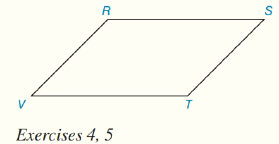Chapter 4.CT, Problem 4CTElementary Geometry For College St...

7th Edition
Alexander + 2 others
ISBN: 9781337614085

Solutions

Chapter
SectionElementary Geometry For College St...

7th Edition
Alexander + 2 others
ISBN: 9781337614085
Textbook Problem

In ▱ R S T V , m ∠ S = 57 ° . Which diagonal ( V S ¯  or  R T ¯ ) would have greater length?To determine

To Find:

Which diagonal has a greater length?

Explanation

Given parallelogram is RSTV,

.

From the property consecutive angles in a parallelogram are supplementary.

Now, from the figure,

T is consecutive to S and,

T is supplementary to S.

Now the sum of measure of two supplementary angles is 180o.

mT+mS=180°mT=180°mS

Still sussing out bartleby?

Check out a sample textbook solution.

See a sample solution

The Solution to Your Study Problems

Bartleby provides explanations to thousands of textbook problems written by our experts, many with advanced degrees!

Get Started

For the following scores, find the value of each expression: X 3 2 4 2 a. X b. (X)2 c. X 2 d. (X 2)

Essentials of Statistics for The Behavioral Sciences (MindTap Course List)

Find y and y. y = ln(l + ln x)

Calculus: Early Transcendentals

Evaluate the integrals in Problems 1-32. Identify the formula used. 27.

Mathematical Applications for the Management, Life, and Social Sciences

(13)0

Applied Calculus for the Managerial, Life, and Social Sciences: A Brief Approach

For , an upper bound estimate for s − s6 (where s6 is the sixth the series does not converge

Study Guide for Stewart's Single Variable Calculus: Early Transcendentals, 8th

In Exercises 57-62, Use Venn diagram to illustrate each statement. A(BC)=(AB)C

Finite Mathematics for the Managerial, Life, and Social Sciences